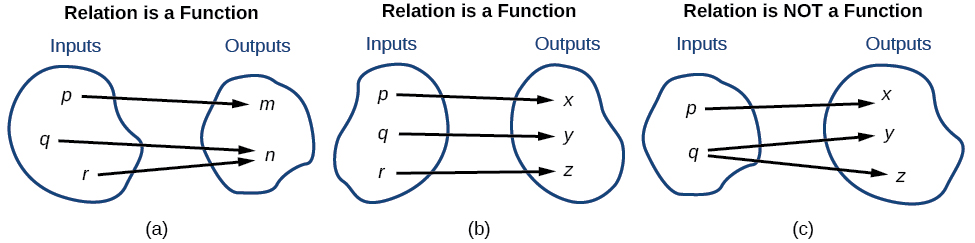# 1.1 Functions and function notation

 Page 1 / 21
In this section, you will:
• Determine whether a relation represents a function.
• Find the value of a function.
• Determine whether a function is one-to-one.
• Use the vertical line test to identify functions.
• Graph the functions listed in the library of functions.

A jetliner changes altitude as its distance from the starting point of a flight increases. The weight of a growing child increases with time. In each case, one quantity depends on another. There is a relationship between the two quantities that we can describe, analyze, and use to make predictions. In this section, we will analyze such relationships.

## Determining whether a relation represents a function

A relation    is a set of ordered pairs. The set of the first components of each ordered pair is called the domain and the set of the second components of each ordered pair is called the range . Consider the following set of ordered pairs. The first numbers in each pair are the first five natural numbers. The second number in each pair is twice that of the first.

$\left\{\left(1,\text{\hspace{0.17em}}2\right),\text{\hspace{0.17em}}\left(2,\text{\hspace{0.17em}}4\right),\text{\hspace{0.17em}}\left(3,\text{\hspace{0.17em}}6\right),\text{\hspace{0.17em}}\left(4,\text{\hspace{0.17em}}8\right),\text{\hspace{0.17em}}\left(5,\text{\hspace{0.17em}}10\right)\right\}$

The domain is $\left\{1,\text{\hspace{0.17em}}2,\text{\hspace{0.17em}}3,\text{\hspace{0.17em}}4,\text{\hspace{0.17em}}5\right\}.$ The range is $\left\{2,\text{\hspace{0.17em}}4,\text{\hspace{0.17em}}6,\text{\hspace{0.17em}}8,\text{\hspace{0.17em}}10\right\}.$

Note that each value in the domain is also known as an input value, or independent variable    , and is often labeled with the lowercase letter $\text{\hspace{0.17em}}x.\text{\hspace{0.17em}}$ Each value in the range is also known as an output value, or dependent variable    , and is often labeled lowercase letter $\text{\hspace{0.17em}}y.$

A function $\text{\hspace{0.17em}}f\text{\hspace{0.17em}}$ is a relation that assigns a single value in the range to each value in the domain . In other words, no x -values are repeated. For our example that relates the first five natural numbers to numbers double their values, this relation is a function because each element in the domain, $\left\{1,\text{\hspace{0.17em}}2,\text{\hspace{0.17em}}3,\text{\hspace{0.17em}}4,\text{\hspace{0.17em}}5\right\},$ is paired with exactly one element in the range, $\left\{2,\text{\hspace{0.17em}}4,\text{\hspace{0.17em}}6,\text{\hspace{0.17em}}8,\text{\hspace{0.17em}}10\right\}.$

Now let’s consider the set of ordered pairs that relates the terms “even” and “odd” to the first five natural numbers. It would appear as

$\left\{\left(\text{odd},\text{\hspace{0.17em}}1\right),\text{\hspace{0.17em}}\left(\text{even},\text{\hspace{0.17em}}2\right),\text{\hspace{0.17em}}\left(\text{odd},\text{\hspace{0.17em}}3\right),\text{\hspace{0.17em}}\left(\text{even},\text{\hspace{0.17em}}4\right),\text{\hspace{0.17em}}\left(\text{odd},\text{\hspace{0.17em}}5\right)\right\}$

Notice that each element in the domain, $\left\{\text{even,}\text{\hspace{0.17em}}\text{odd}\right\}$ is not paired with exactly one element in the range, $\left\{1,\text{\hspace{0.17em}}2,\text{\hspace{0.17em}}3,\text{\hspace{0.17em}}4,\text{\hspace{0.17em}}5\right\}.$ For example, the term “odd” corresponds to three values from the domain, $\left\{1,\text{\hspace{0.17em}}3,\text{\hspace{0.17em}}5\right\}$ and the term “even” corresponds to two values from the range, $\left\{2,\text{\hspace{0.17em}}4\right\}.$ This violates the definition of a function, so this relation is not a function.

[link] compares relations that are functions and not functions.(a) This relationship is a function because each input is associated with a single output. Note that input   q   and   r   both give output   n .   (b) This relationship is also a function. In this case, each input is associated with a single output. (c) This relationship is not a function because input     q   is associated with two different outputs.

## Function

A function    is a relation in which each possible input value leads to exactly one output value. We say “the output is a function of the input.”

The input    values make up the domain    , and the output    values make up the range    .

Given a relationship between two quantities, determine whether the relationship is a function.

1. Identify the input values.
2. Identify the output values.
3. If each input value leads to only one output value, classify the relationship as a function. If any input value leads to two or more outputs, do not classify the relationship as a function.

a colony of bacteria is growing exponentially doubling in size every 100 minutes. how much minutes will it take for the colony of bacteria to triple in size
what is the importance knowing the graph of circular functions?
can get some help basic precalculus
What do you need help with?
Andrew
how to convert general to standard form with not perfect trinomial
can get some help inverse function
ismail
Rectangle coordinate
how to find for x
it depends on the equation
Robert
whats a domain
The domain of a function is the set of all input on which the function is defined. For example all real numbers are the Domain of any Polynomial function.
Spiro
foci (–7,–17) and (–7,17), the absolute value of the differenceof the distances of any point from the foci is 24.
difference between calculus and pre calculus?
give me an example of a problem so that I can practice answering
x³+y³+z³=42
Robert
dont forget the cube in each variable ;)
Robert
of she solves that, well ... then she has a lot of computational force under her command ....
Walter
what is a function?
I want to learn about the law of exponent
explain this
what is functions?
A mathematical relation such that every input has only one out.
Spiro
yes..it is a relationo of orders pairs of sets one or more input that leads to a exactly one output.
Mubita
Is a rule that assigns to each element X in a set A exactly one element, called F(x), in a set B.
RichieRich
If the plane intersects the cone (either above or below) horizontally, what figure will be created?ByBy Eddie UnverzagtBy Brooke DelaneyBy OpenStaxBy Edward BitonBy Richley CrapoBy RhodesBy Jonathan LongBy OpenStaxBy OpenStaxBy Bonnie Hurst